Learn about hash table, hash function, hash code, its implementation and running time.

# Hash Table

• Hash table: a data structure that maps keys to values for highly efficient lookup.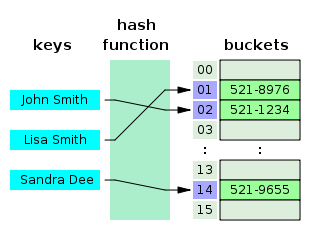• Hash function: to map general keys to corresponding indices in a table
• The goal of a hash function is to map each key $k$ to an integer in the range $[0,N-1]$.
• Two parts of a hash function: a hash code and a compression function
• Hash code: maps a key $k$ to an integer
• Compression function: maps the hash code to an integer within a range of indices for a bucket array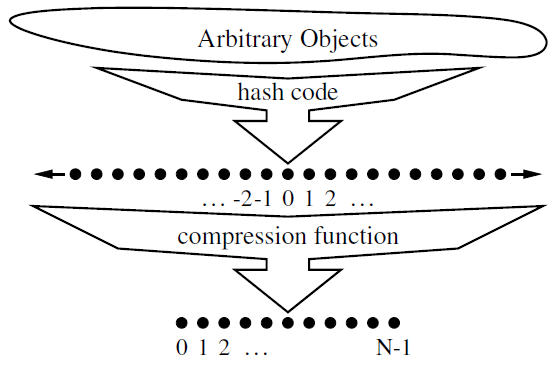• Bucket array: an array where each index obtains a bucket of collection of items for two different keys with the same index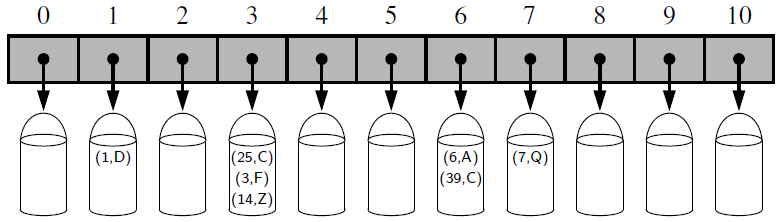• Collision: if there are two or more keys with the same hash value, then two different items will be mapped to the same bucket and a collision has occurred.

# Implementation

• For the simple implementation, we use an array of linked lists and a hash code function.
1. Compute the key’s hash code (Two different keys could have the same hash code)
2. Map the hash code to an index in the array (Two different hash codes could map to the same index)
3. Store the key and value in the index. (Use linked list because of collision)
• To retrieve the value pair by its key, repeat the same process
1. Compute the hash code from the key
2. Compute the index from the hash code
3. Search through the linked list for the value with this key

# Running Time

• Comparison of the running times between unsorted list and hash table where $n$ denote the number of items in the map.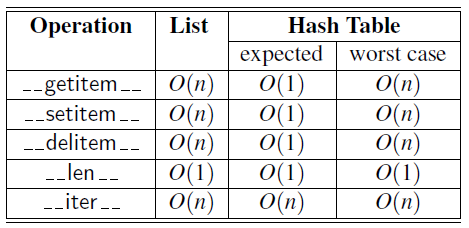• If the number of collisions is very high, the worst case is $O(n)$ and it is $O(1)$ for minimum collisions.
• Alternatively, hash table can be implemented with a balanced binary search tree.
• It gives $O(\log N)$ lookup time.
• It potentially uses less space and is able to iterate through the keys in order, which can be useful sometimes.
• Time complexity of dictionary in Python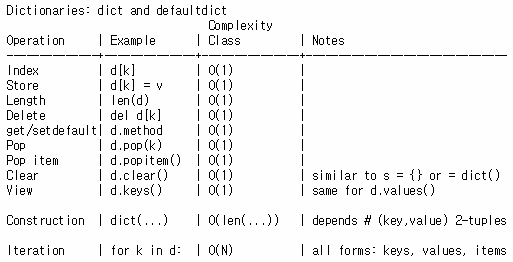# Quiz

• Is Double: Find the first recurring character in a string.

# Naive way takes O(n^2)
def isDouble_naive(str):
# For each 'i'th char, we check whether it occurs double
for i in range(len(str)):
for j in range(i+1, len(str)):
if str[i] == str[j]:
return str[i]
return None


## Better way using ‘dictionary’

# Better way using dict takes O(n)
def isDouble_dict(str):
# Using dictionary (hash table)
ht = dict()
for c in str:
if c not in ht: # O(1) for average case, O(n) for worst case
ht[c]=1 # O(1)
else:
return c
return None


## Better way using ‘set’

# Better way using set takes O(n)
def isDouble_set(str):
# Using dictionary (hash table)
ht = set()
for c in str:
if c not in ht: # O(1) for average case, O(n) for worst case
else:
return c
return None


# Quiz

• Is Unique: Implement an algorithm to determine if a string has all unique characters. What if you cannot use additional data structures?

def isunique_naive(string):
for i in range(len(string)):
if string.find(string[i], i+1) != -1:
return False # Char is not unique
return True # All unique characters


# O(N)
def isunique(string):
# Assuming character set is ASCII (128 characters)
if len(string) > 128:
return False

char_set = [False for _ in range(128)]
for char in string:
val = ord(char) # Char to ASCII
if char_set[val]:
return False # Char already found in string
char_set[val] = True

return True

• Book: Cracking the coding interview [Link]
• Book: Data structures and algorithms in python [Link]